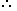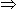# Number Problems

Q1
A number consists of two digits. If the digits interchange places and the new number is added to the original number, then the resulting number will be divisible by?
Ans:

Let the ten's digit be x and unit's digit be y.

Then, number = 10x + y.

Number obtained by interchanging the digits = 10y + x.(10x + y) + (10y + x) = 11(x + y), which is divisible by 11.

Q2

In a two-digit, if it is known that its unit's digit exceeds its ten's digit by 2 and that the product of the given number and the sum of its digits is equal to 144, then the number is?

Ans:

Let the ten's digit be x.

Then, unit's digit = x + 2.

Number = 10x + (x + 2) = 11x + 2.

Sum of digits = x + (x + 2) = 2x + 2.(11x + 2)(2x + 2) = 14422x2 + 26x - 140 = 011x2 + 13x - 70 = 0(x - 2)(11x + 35) = 0x = 2.

Hence, required number = 11x + 2 = 24.

Q3

The product of two numbers is 9375 and the quotient, when the larger one is divided by the smaller, is 15. The sum of the numbers is?

Ans:

Let the numbers be x and y.

Then, xy = 9375 and x/y=15

xy/(x/y) = 9375/15y = 25.y2 = 625.x = 15y = (15 x 25) = 375.Sum of the numbers = x + y = 375 + 25 = 400.

Q4

The product of two numbers is 120 and the sum of their squares is 289. The sum of the number is?

Ans:

Let the numbers be x and y.

Then, xy = 120 and x2 + y2 = 289.(x + y)2 = x2 + y2 + 2xy = 289 + (2 x 120) = 529x + y = 529 = 23.

Q5

What is the sum of two consecutive even numbers, the difference of whose squares is 84?

Ans:

Let the numbers be x and x + 2.

Then, (x + 2)2 - x2 = 844x + 4 = 844x = 80x = 20.The required sum = x + (x + 2) = 2x + 2 = 42.

Q6

The sum of two number is 25 and their difference is 13. Find their product?
Ans:

Let the numbers be x and y.

Then, x + y = 25 and x - y = 13.

4xy = (x + y)2 - (xy)2

= (25)2 - (13)2

= (625 - 169)

= 456xy = 114.